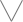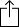C
D
E
F
G
A
B
Chord
Scale
Custom
3nps
CAGED
None
Notes
Degrees
Intervals
None

1
2
3
4
5
6
7
1
2
3
4
5
6
7
8
9
10
11
12
13
14
15
16
17
18
19
20
21
22
23
24
E
B
G
D
A
E
F
F
G
A
A
A
B
C
C
D
E
E
F
F
G
A
A
A
B
C
C
D
E
E
C
C
D
E
E
F
F
G
A
A
A
B
C
C
D
E
E
F
F
G
A
A
A
B
A
A
A
B
C
C
D
E
E
F
F
G
A
A
A
B
C
C
D
E
E
F
F
G
E
E
F
F
G
A
A
A
B
C
C
D
E
E
F
F
G
A
A
A
B
C
C
D
A
B
C
C
D
E
E
F
F
G
A
A
A
B
C
C
D
E
E
F
F
G
A
A
F
F
G
A
A
A
B
C
C
D
E
E
F
F
G
A
A
A
B
C
C
D
E
E

D Ionian #5 for Guitar - 3nps Chart

D Ionian #5 is a diatonic scale and is the 3rd mode in the set of Harmonic minor modes

D
E
F
G
A
B
C
DW
W
H
aug2
H
W
H
Play scaleand then